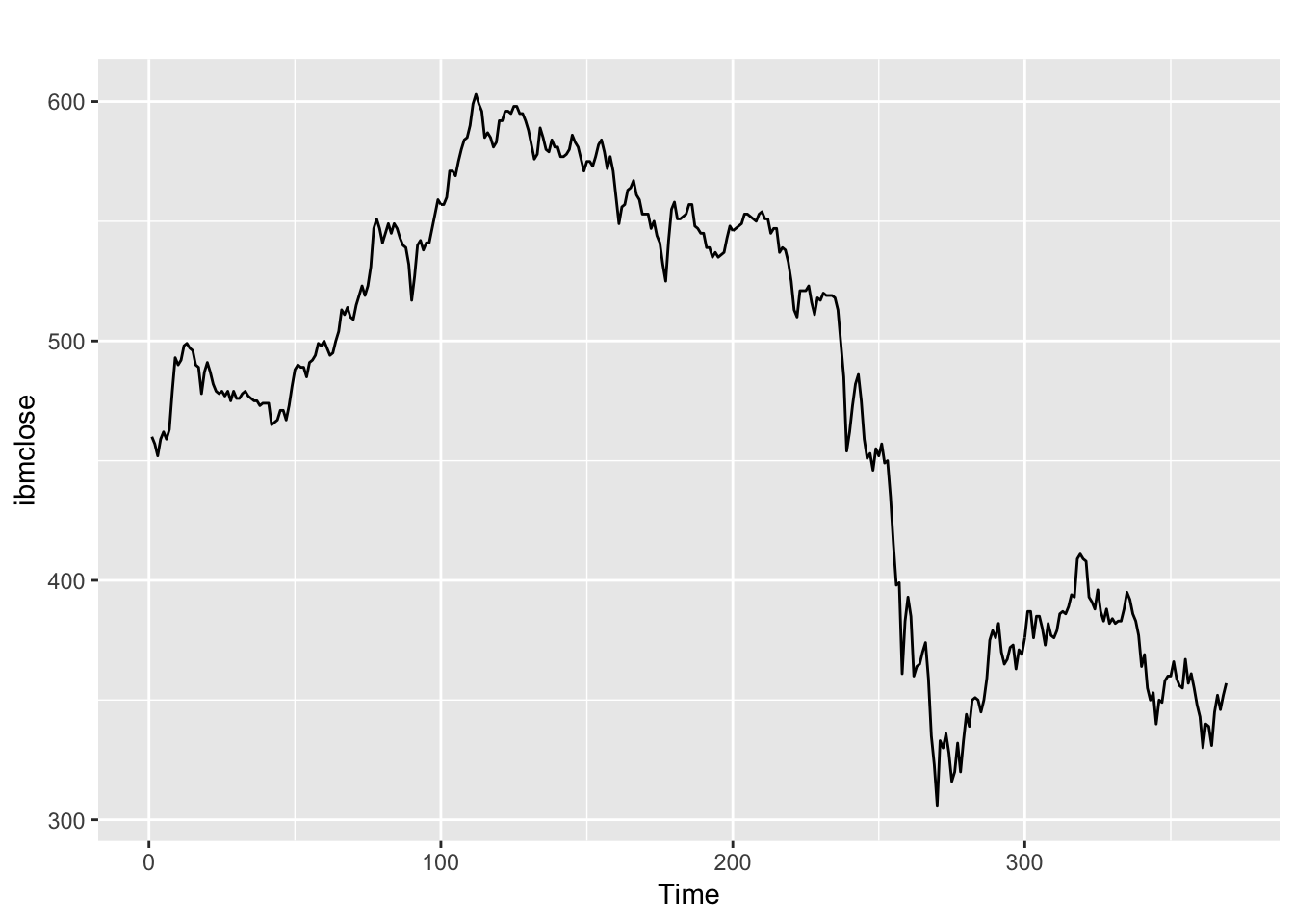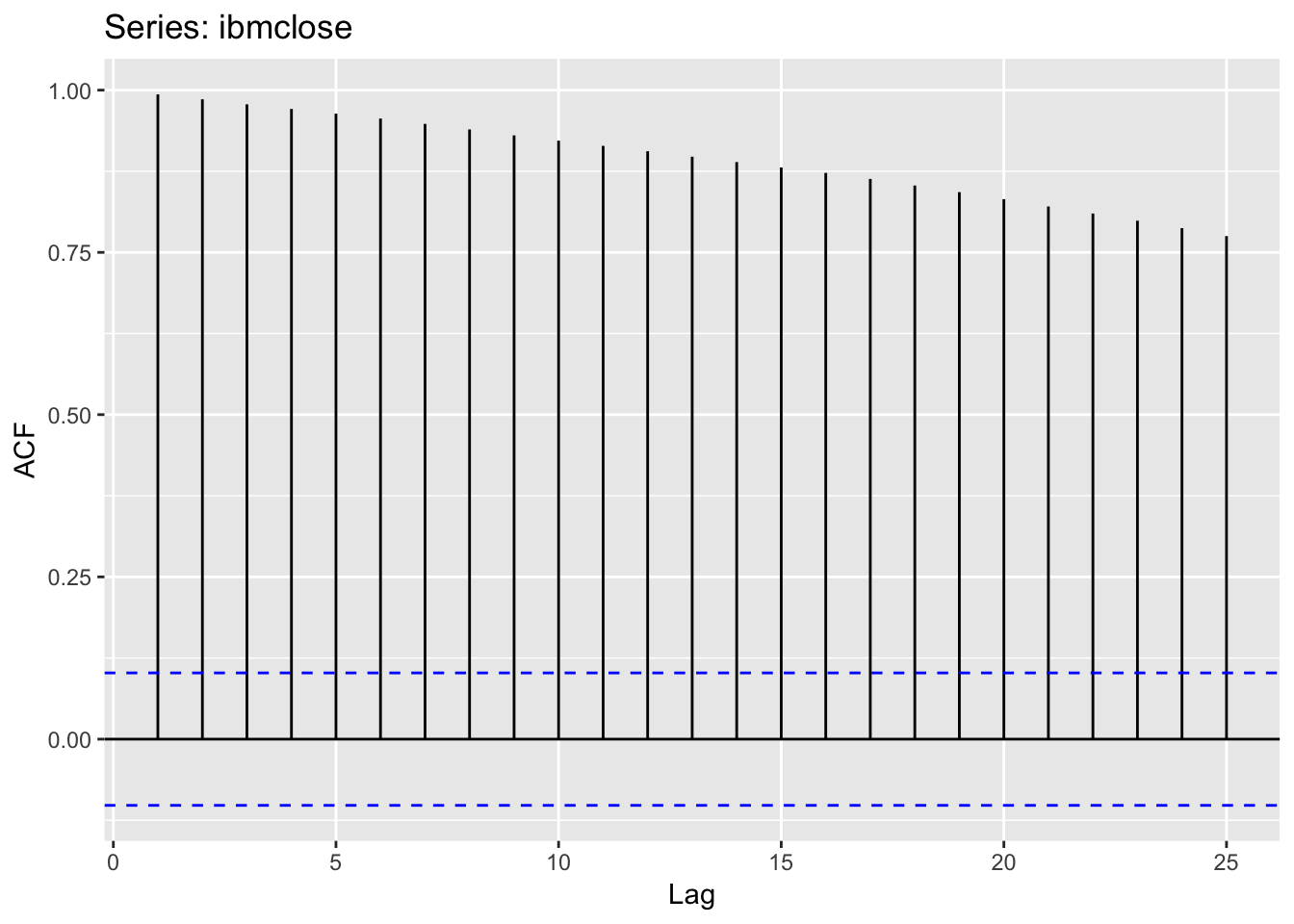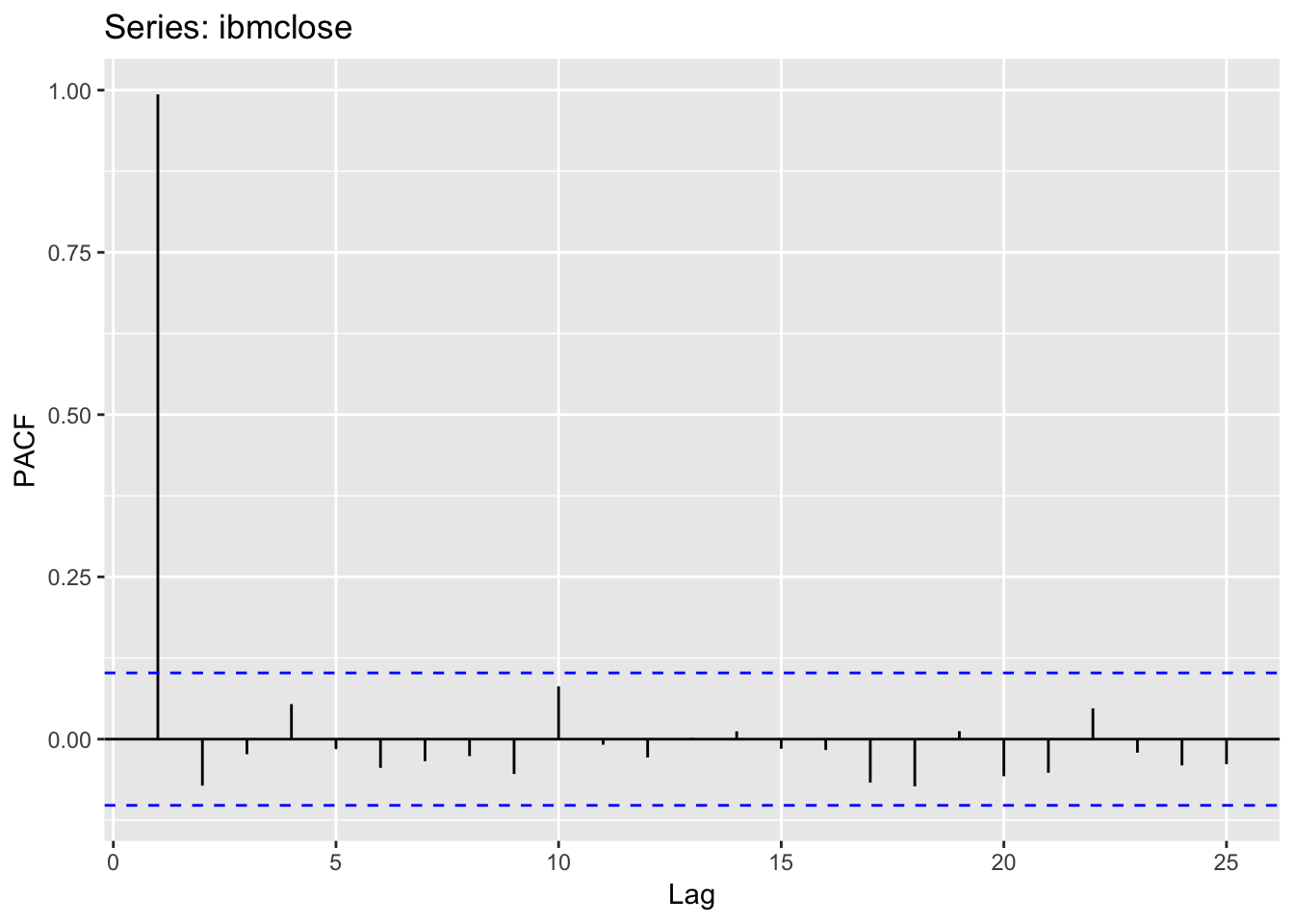### Exercise 8.1

Figure 8.31 shows the ACFs for 36 random numbers, 360 random numbers and 1,000 random numbers.

#### a)

Explain the differences among these figures. Do they all indicate that the data are white noise?

ACFs (autocorrelation functions) are plotted for random numbers series of various size (36, 360, and 1000). All ACFs are indicating that the data is white noise since the dashed blue lines indicate whether the correlations are significantly different from zero and for all three plots that is the case.

#### b)

Why are the critical values at different distances from the mean of zero? Why are the autocorrelations different in each figure when they each refer to white noise?

The critical values are getting smaller as the size of our series gets larger. According to chapter 2.9 of the textbook: “For a white noise series, we expect 95% of the spikes in the ACF to lie within ±2/√T where T is the length of the time series.” Basically a larger our T is - the smaller our critical values will be.

### Exercise 8.2

A classic example of a non-stationary series is the daily closing IBM stock price series (data set ibmclose). Use R to plot the daily closing prices for IBM stock and the ACF and PACF. Explain how each plot shows that the series is non-stationary and should be differenced.

``data(ibmclose)``
``## Warning in data(ibmclose): data set 'ibmclose' not found``
``autoplot(ibmclose)````ggAcf(ibmclose)````ggPacf(ibmclose)``The plot of the data has both changing trend and levels which indicate that the series is non-stationary. The ACF plot also confirms this - as ACF of non-stationary data decreases slowly, and the value of r1 is often large and positive. The PACF indicates a strong correlation between the data and the first lag. Differencing can help stabilise the mean of this time series by removing changes in the level of a time series.

### Exercise 8.3

For the following series, find an appropriate Box-Cox transformation and order of differencing in order to obtain stationary data.

#### usnetelec

``autoplot(usnetelec)``